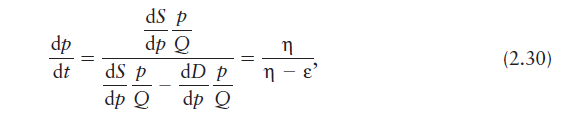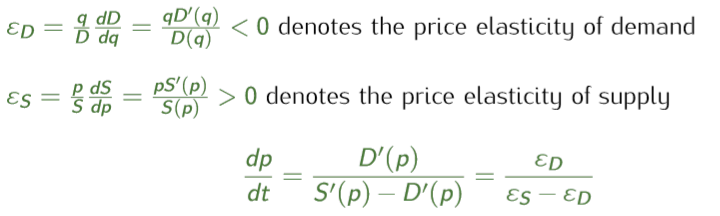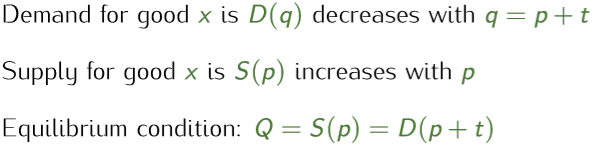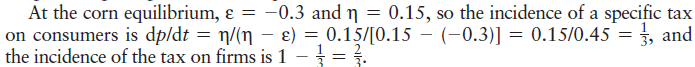# Tax Incidence formulas in the view of producer and consumer

My daughter attends her first college class, Micro economy, at the summer of her junior year. So, it is time to go over some economy concepts that I learnt before to prepare questions from daughter.

Get the textbook from Prof. Jeffrey M. Perloff – Microeconomics_ Theory and Applications with Calculus (Pearson, 4th) Of course, this textbook full of math formula is different than my daughter’s economy class. But I always prefer math formula than the description.

When reading the tax incidence formula in the textbook, it is different than the formula mentioned in Harvard’s’ economy class lecture ppt, https://scholar.harvard.edu/files/stantcheva/files/lecture3.pdf.  Of course, as a no-name, I have to believe those two formulas are identity.  So, it is time for me to get the root why they are all correct.

1. The Problem:

Ok. Here are the two different formulas in Prof. Jeffrey M. Perloff textbook and Harvard’s’ lecture ppt.

1. Textbook (4th version): P66, formula 2.301. Harvard’s’ lecture ppt: P9, the top formulaIf we use the same symbol of elastics, the final formula will be as belowSo, it is easy to throw a questions: The two equations are not the same if read it verbatim, as denominators in both formula right side are the same, but nominators are different.  But why the two formulas have the same left side? Seems only one and just one of the formulas is correct, and there is no chance that both formulas are correct.  For example, A=1 and A=2.  You can’t say A is ok to 1 and 2.

2. The Explanation:

Actually, the two formulas are both correct. That is because the definition of left side are different.  The textbook deduced the formula from the consumer’s view, while the lecture ppt got its from the producer’s view.

In the consumer’s view (textbook) formula is deduced from formula,; While in the producer’s view (lecture) formula is from below formula(the last one)From the above two original source, here is the discovery. In the consumer’s view source formula, the real market equilibrium price is p, the tax t is collected by producer and producer earns p-t; however, in the producer’s view source formula, the equilibrium price is p+t, and the producer earns p and consumer paid the tax t by themselves later.

So, back to theformula. The textbook formula left sidemeans what is the customer paid change when tax price change. While in lecture formula left side, it actually say what is the producer earns’ change when tax price change.

As the definitions ofare different, it is ok that the right side of the two equations are different. But further, we must prove that the result of the tax incidence on consumer and producer should be the same when using the two different formulas. Here we will use both math deduction and example to test it.

3. The Tax Incident Math Deduction

First, we know that the demand curve is down when price is up and supply curve is adverse. So, we gotIn the consumer’s view with:

We got.  So, the consumer pays additionalwith tax t added. That is the tax incidence on consumer side.

The producer’s earns q is p-t, so the change is. That means the producer will have less earning. The less amountis actually the tax incidence on producer side. The producer’s earns change formula is same as the formula in the producer view.

Of course, you can also prove it with the producer view direction. We will ignore it in the article.

4. The Tax Incident Example

Let’s use the textbook example in P66 as below:The textbook example already calculates the result in the consumer’s view. Assumed the tax changeis \$1, the additional pricethat the customer pays is; while the firm (producer) will pay that additional. So that, the total tax;

Let’s use producer’s view formula,. Assumed the same tax change (), the producer earns’ change is.  That means the producer will pay the tax incidence. So the remainingwill be paid by consumers.

The tax incidence result are same in both formulas.